# Reasoning for SSC CHSL/CGL 15th January 2020 for figure counting and mirror image

Q1. Find the number of triangles in the given figure?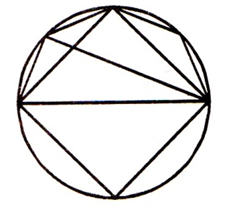(a) 8
(b) 10
(c) 11
(d) 12

Q2. In the following questions, choose the correct mirror image of figure X, from the given alternatives.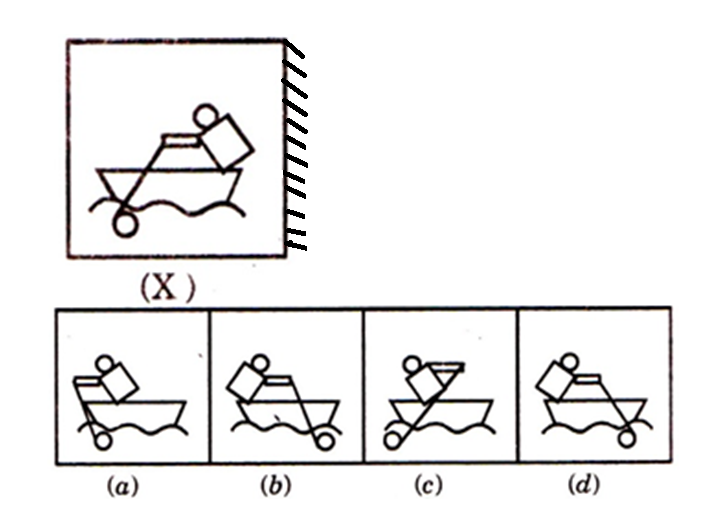(a) a
(b) b
(c) c
(d) d

Q3. Find the water image of the given of the given combination.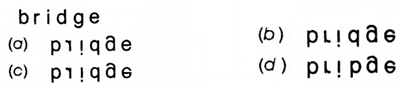(a) a
(b) b
(c) c
(d) d

Q4. Find the alternative figures which contains figure (X)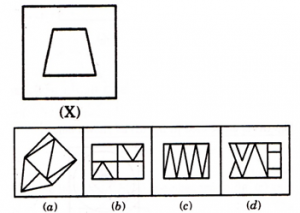(a) a
(b) b
(c) c
(d) d

Q5. Select a figure from amongst the four alternative, which when placed in the black space of figure (X) would complete the pattern.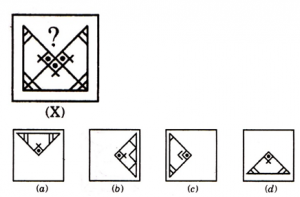(a) a
(b) b
(c) c
(d) d

Q6. Choose a figure from the given alternatives, which would most closely resemble the unfolded form of fig (Z).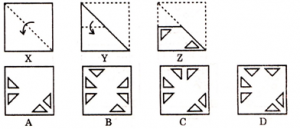(a) A
(b) B
(c) C
(d) D

Q7. In the following question, group the given figures into three classes using each figure only once.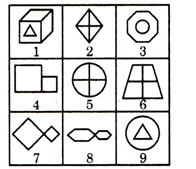(a) 1, 3, 9 ; 2, 5, 6 ; 4, 7, 8
(b) 1, 3, 9 ; 2, 7, 8 ; 4, 5, 6
(c) 1, 2, 4 ; 3, 5, 7 ; 6, 8, 9
(d) 1, 3, 6 ; 2, 4, 8 ; 5, 7, 9

Q8. How many dots lie opposite the face having three dots, when the given figure is folded to form a cube?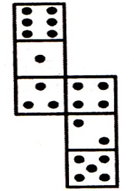(a) 2
(b) 4
(c) 5
(d) 6

Q9. When decoded, OPTRRE reads as PORTER, In the same way, what does the following read as ?

EROPTR

(a) ROPE
(b) PROPER
(c) PORT
(d) REPORT

Q10. If TYPEWRITER is written as GBKVDIRGVI, how STENO can be written in that code?

(a) LMVGH
(b) HGVML
(c) LMHGV
(d) HVLGM

#### Solutions:

S1. Ans.(b)
Sol.
There are 10 triangles.

S2. Ans.(d)

S3. Ans.(b)

S4. Ans.(c)

S5. Ans.(d)

S6. Ans.(c)

S7. Ans.(a)

S8. Ans.(d)
Sol.
Opposite faces are :-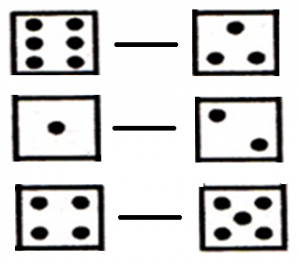S9. Ans.(d)
Sol.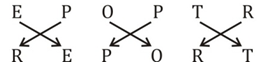S10. Ans.(b)
Sol.
Code is generated by using opposite letters.

##### SSC CGL | SSC CHSL 2020 | Free Marathon Class English, Science, Maths, Reasoning

You may also like to read: